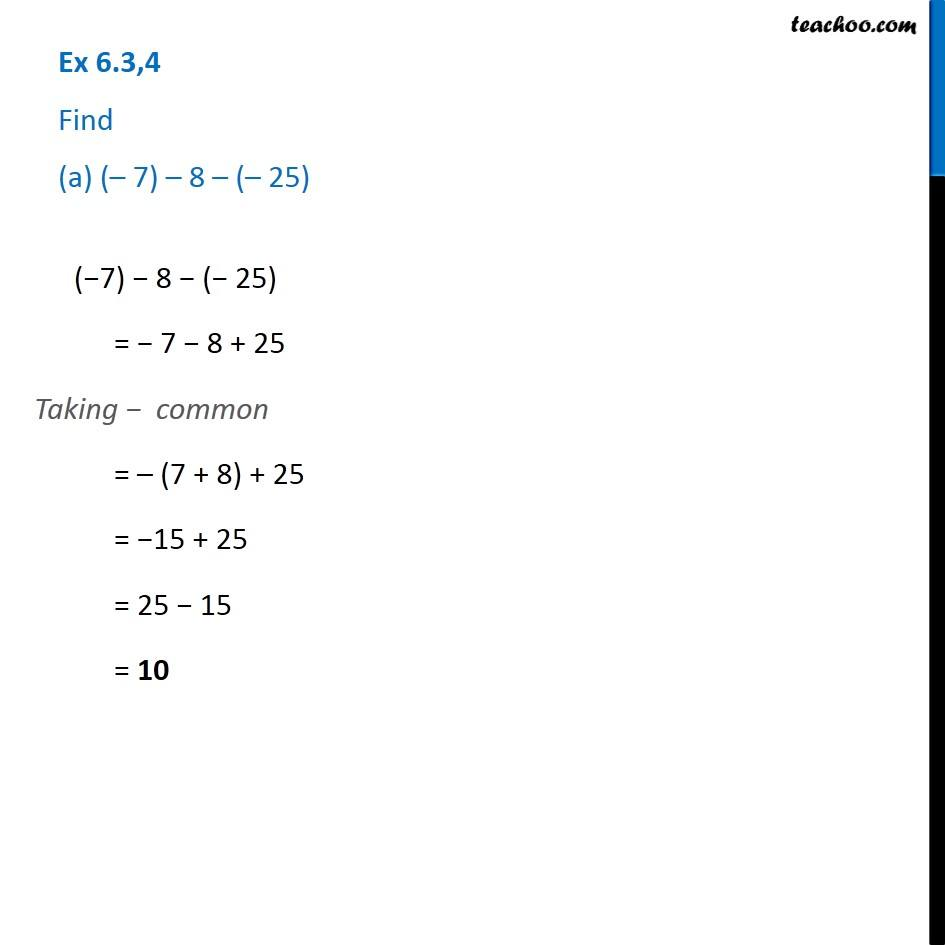1. Chapter 6 Class 6 Integers
2. Serial order wise
3. Ex 6.3

Transcript

Ex 6.3,4 Find (a) (– 7) – 8 – (– 25) (−7) − 8 − (− 25) = − 7 − 8 + 25 Taking − common = – (7 + 8) + 25 = −15 + 25 = 25 − 15 = 10

Ex 6.3